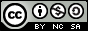- 目录 -
LeetCode 337 打家劫舍 III（树形dp）

输入: [3,2,3,null,3,null,1]

3
/ \
2   3
\   \
3   1



输入: [3,4,5,1,3,null,1]

3
/ \
4   5
/ \   \
1   3   1



## 思路

• dp[node] 代表以 node为根，不选 node 这个点可以取到的最大权值。
• dp[node] 代表以 node为根，选择 node 这个点可以取到的最大权值。

• $dp[node]=max(dp[son],dp[son])$ 当前点不选，则儿子可以选也可以不选
• $dp[node]=dp[node]+dp[son]$ 选择当前点，则儿子点不能选

• $dp[node]=0$
• $dp[node]=val$

## 代码

  1 2 3 4 5 6 7 8 9 10 11 12 13 14 15 16 17 18 19 20 21 22 23 24 25 26 27 28 29 30 31 32 33 34 35 36 37 38 39 40 41 42 43 44 45 46 47  struct TreeNode { int val; TreeNode *left; TreeNode *right; TreeNode(int x) : val(x), left(NULL), right(NULL) {} }; class Solution { public: unordered_map dp0, dp1; void dfs(TreeNode *node) { if (node->left) { dfs(node->left); dp0[node] += max(dp0[node->left], dp1[node->left]); dp1[node] += dp0[node->left]; } if (node->right) { dfs(node->right); dp0[node] += max(dp0[node->right], dp1[node->right]); dp1[node] += dp0[node->right]; } } int rob(TreeNode *root) { if (!root) return 0; queue q; q.push(root); while (!q.empty()) { TreeNode *node = q.front(); q.pop(); dp1[node] = node->val; if (node->left) q.push(node->left); if (node->right) q.push(node->right); } dfs(root); return max(dp0[root], dp1[root]); } };